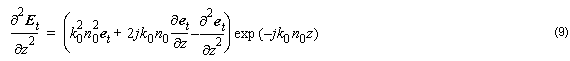## E-Formulation

The full vectorial wave equation is given by: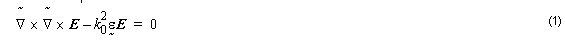Considering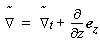into Equation 1, we get: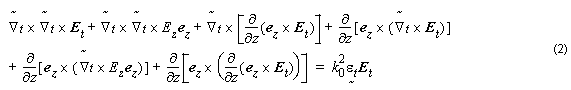The term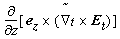is null.

We can separate Equation 2 into two equations, one for longitudinal terms and another for transversal terms: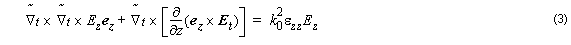for longitudinal terms and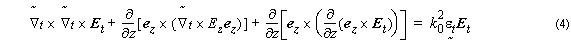for the transversal terms.
By substituting Equation 3 into the first right hand term of Equation 4, we get: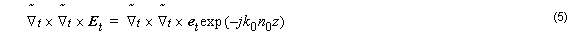We can write: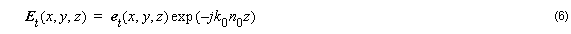By substituting Equation 6 into the second right hand term of Equation 4, we get: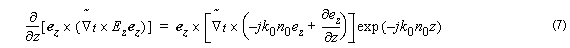The third term can be rewritten as: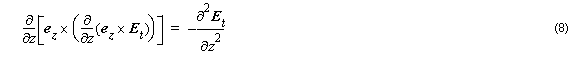Now, by using Equation 5 in the left side of Equation 8, we get: